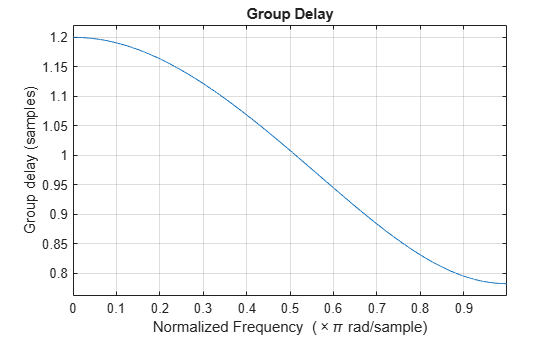fdesign.fracdelay

Fractional delay filter specification object

Syntax

d = fdesign.fracdelay(delta)
d = fdesign.fracdelay(delta,'N')
d = fdesign.fracdelay(delta,'N',n)
d = fdesign.fracdelay(delta,n)
d = fdesign.fracdelay(...,fs)

Description

d = fdesign.fracdelay(delta) constructs a default fractional delay filter designer d with the filter order set to 3 and the delay value set to delta. The fractional delay delta must be between 0 and 1 samples.

d = fdesign.fracdelay(delta,'N') initializes the filter designer specification to N, where N specifies the fractional delay filter order and defaults to filter order of 3.

Use designmethods(d) to get a list of the design methods available for a specification.

d = fdesign.fracdelay(delta,'N',n) initializes the filter designer to N and sets the filter order to n.

d = fdesign.fracdelay(delta,n) assumes the default specification N, filter order, and sets the filter order to the value you provide in input n.

d = fdesign.fracdelay(...,fs) adds the argument fs, specified in units of Hertz (Hz) to define the sampling frequency. In this case, specify the fractional delay delta to be between 0 and 1/fs.

Examples

collapse all

Design a second-order fractional delay filter of 0.2 samples using the Lagrange method. Implement the filter using a Farrow fractional delay (fd) structure.

d = fdesign.fracdelay(0.2,'N',2);
secondOrderFrac = design(d,'lagrange','filterstructure','farrowfd');
fvtool(secondOrderFrac,'analysis','grpdelay')Design a cubic fractional delay filter with a sampling frequency of 8 kHz and fractional delay of 50 microseconds using the Lagrange method.

d = fdesign.fracdelay(50e-6,'N',3,8000);
cubicFrac = design(d, 'lagrange', 'FilterStructure', 'farrowfd');